Search Results (2228)

View
Selected filters:
• MathematicsConditions of Use:
Remix and Share
Rating

Subject:
Mathematics
Material Type:
Unit of Study
Provider:
OS/MAISA
03/22/2018Conditions of Use:
Remix and Share
Rating

The focus of this unit is to develop students' fluency with addition and subtraction facts within 10. To do this, students continue to work with number patterns and relationships, including skip counting by 5s and 10s and identifying the relationship between written and spoken number words and written numerals. They order and compare numbers to develop an understanding of their relative sizes. They become more skilled at instantly recognizing the amounts in a patterned set of objects without counting them (subitizing), e.g., dots on dice, dominos, or a ten-frame. These activities engage students in thinking about part-part-total number relationships and aid in learning the number combinations foundational to learning other basic addition and subtraction facts in first grade.Students compose and decompose numbers to ten, which provides experiences with the big mathematical ideas of equivalence and the commutative property for addition. They also learn to use strategies such as adjusting the numbers in a problem to make it easier to solve (e.g., 6 + 4 = 5 + 5; 2 + 4 = 3 + 3). They develop fluency with complements of ten to establish ten as an anchor or benchmark number for future work with addition and subtraction. They solve different types of word problems within sums of 10 that include concretely, pictorially and numerically modeling and explaining their solutions.When reading the standards below, keep in mind that the focus of this unit is developing strategies and fluency for adding and subtracting within 10. Students will work with larger numbers later in the year.

Subject:
Mathematics
Material Type:
Unit of Study
Provider:
OS/MAISA
03/22/2018Conditions of Use:
Remix and Share
Rating

The focus of this unit is on deepening students’ use of mental strategies for facts as fluency with basic addition and subtraction facts is foundational for multi-digit computation. In first grade students explored a variety of reasoning strategies for finding the sum of two one-digit numbers. In second grade students need to become fluent with basic faction order to free up more complex problems including two- and three-digit addition and subtraction problems. As students work to deepen and extend their strategies for facts above ten, they work with place value concepts. The work students do with place value in this unit reviews decomposing numbers into tens and ones. As students explain their thinking and listen to the thinking of others, they expand their understanding of number relationships and properties of operations. For example, one fact strategy for adding 7 + 9 is to think, “7 + 9 = (6 + 1) + 9 = 6 + (1 + 9) = 6 + 10 = 16.” Another strategy for 7 + 9 is to think, “7 + 9 = 7 + 10 - 1 = 17 – 1 = 16.” These are useful strategies that students may also apply when using mental arithmetic to add 8 or 9 ones to a multi-digit number (e.g., 46 + 8 = 8 + 2 + 44 = 10 + 44 = 54 or 46 +10 – 2 = 56 – 2 = 54).

Subject:
Mathematics
Material Type:
Unit of Study
Provider:
OS/MAISA
03/22/2018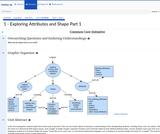Conditions of Use:
Remix and Share
Rating

To develop “spatial sense”, students use positional words to describe the location of physical objects in the classroom or school (above, below, beside, in front of, behind, next to).

Subject:
Mathematics
Material Type:
Unit of Study
Provider:
OS/MAISA
03/22/2018Conditions of Use:
Remix and Share
Rating

In this unit, students build the foundational understandings that functions model relationships between quantities. In addition, students build an arsenal of tools to study functions throughout the course including the routine of examining functions with multiple representations. “Students should develop ways of thinking that are general and allow them to approach any type of function, work with it, and understand how it behaves, rather than see each function as a completely different animal in the bestiary” (Grade 8, High School Functionsprogression document, page 7). Specific features of linear, exponential, quadratic, and polynomial functions are each studied extensively in their own units in Algebra 1. Many standards that are introduced in Unit 1 will be further developed and/or utilized across these future units; look for these standards under the Unit Level Standards heading.Contexts are important for developing conceptual understanding. Drawing from contexts, students justify that one quantity depends on another and that each element of the domain corresponds to exactly one element of the range. They can qualitatively describe aspects of functions (e.g., increasing, decreasing) and reason about a function’s domain and range leading to conjectures about additional representations. In addition, students use contexts to make sense of function notation. For example, if the functionhrepresents the height in centimeters of a bean sprout at specified days,t, then students should be able to talk about and/or identify each of the following:h(3), 5=h(t), h(6.5)=13.2,andh(t)=n.When functions are expressed symbolically, students input values into the equations to generate a table of output values and use these corresponding values to graph the function. After manually generating numeric and then graphic representations, students will use technologies such as a graphing calculator to generate both tables of values and graphs of functions. In doing so, they should pause to reflect upon the functional relationship between variables exhibited in these representations and why the representations make sense. By generating and comparing multiple cases, students recognize that functions can be organized by similar and different features like patterns of change, restrictions in the domain/range, and general shape. This organization of functions both serves as as an introduction to the families of functions that they will study throughout the course and equips students with strategies to study and represent functions.Students should not be expected to generate symbolic representations until later units. Likewise, symbolic manipulation of equations to reveal key features of functions (e.g., intercepts, maximum, horizontal asymptote) is included later in specific function units.

Subject:
Mathematics
Material Type:
Unit of Study
Provider:
OS/MAISA
03/22/2018Conditions of Use:
No Strings Attached
Rating

This is a task from the Illustrative Mathematics website that is one part of a complete illustration of the standard to which it is aligned. Each task has at least one solution and some commentary that addresses important asects of the task and its potential use. Here are the first few lines of the commentary for this task: First pose the question: Here are four triangles. What do all of these triangles have in common? What makes them different from the figures that are no...

Subject:
Mathematics
Material Type:
Activity/Lab
Provider:
Illustrative Mathematics
Provider Set:
Illustrative Mathematics
Author:
Illustrative Mathematics
05/24/2013Conditions of Use:
No Strings Attached
Rating

This is a task from the Illustrative Mathematics website that is one part of a complete illustration of the standard to which it is aligned. Each task has at least one solution and some commentary that addresses important asects of the task and its potential use. Here are the first few lines of the commentary for this task: Materials * A copy of Grandfather Tang's Story by Ann Tompert * One set of tangrams for each student (see note in commentary) * A set of tangrams for t...

Subject:
Mathematics
Material Type:
Activity/Lab
Provider:
Illustrative Mathematics
Provider Set:
Illustrative Mathematics
Author:
Illustrative Mathematics
05/29/2013Conditions of Use:
Remix and Share
Rating

The need to reason logically occurs daily. Principles of reasoning are useful whether developing a strategy to win a game or the sequence of steps needed to repair a lamp. Students use coordinates, diagrams including constructions, and language (i.e. precise terms and conditional statements) to solve problems in this unit and establish them as tools to use in reasoning throughout the course., students will move from explaining their reasoning informally to more formal explanations. Using valid arguments and reasoning patterns with correct assumptions and careful explanation of the reasoning is important. Students build flexibility with the forms used to prove theorems. Paragraph proofs, flow chart proof, two-column proofs, transformational proofs, coordinate proofs, arguments affirming the hypothesis, chains of if-then statements, and use of counterexamples are some possible methods students could use to prove a conjecture.

Subject:
Mathematics
Material Type:
Unit of Study
Provider:
OS/MAISA
03/22/2018Conditions of Use:
No Strings Attached
Rating

This is a task from the Illustrative Mathematics website that is one part of a complete illustration of the standard to which it is aligned. Each task has at least one solution and some commentary that addresses important asects of the task and its potential use. Here are the first few lines of the commentary for this task: Materials - 2 clear plastic cups for each pair of students - 4 bean seeds for each pair - soil - unifix cubes - a plant or math journal to record data ...

Subject:
Mathematics
Material Type:
Activity/Lab
Provider:
Illustrative Mathematics
Provider Set:
Illustrative Mathematics
Author:
Illustrative Mathematics
04/17/2013Conditions of Use:
No Strings Attached
Rating

This is a task from the Illustrative Mathematics website that is one part of a complete illustration of the standard to which it is aligned. Each task has at least one solution and some commentary that addresses important asects of the task and its potential use. Here are the first few lines of the commentary for this task: Materials * Completed monthly weather recording sheet * Crayons * Sentence strips with frames (see below) * Student worksheet Actions Every day for a m...

Subject:
Education
Mathematics
Material Type:
Activity/Lab
Provider:
Illustrative Mathematics
Provider Set:
Illustrative Mathematics
Author:
Illustrative Mathematics
11/05/2013Conditions of Use:
Remix and Share
Rating

This unit extends what students learned in third grade regarding multiplication and division by developing more precise use of mathematical vocabulary and more sophisticated ways of analyzing number patterns (see 4.OA.C.5.). As part of their analysis of number patterns students deepen their understanding of the structure of the base ten system paying particular attention to how the value of a digit changes given its position in a number (e.g., in the number 7,700 the value of the 7 in the thousands place is ten times that of the 7 in the hundreds place). They also notice that numbers have unique characteristics such as prime, composite, and square and they begin to understand why these characteristics can be important when solving problems. Students develop mathematical vocabulary as a tool to describe and reason about the multiplication and division equations they write and solve (e.g., factor, divisor, multiple, quotient). They are an understanding of prime, composite, and square numbers and begin to understand why these are helpful ways to describe numbers . They also describe equations by referencing the numbers the Precise mathematical language includes such terms as equations, factors, product, quotient, multiples, prime, composite and square as they apply to numerical attributes. While the ideas developed in this unit In earlier grades, students use multiplication facts to compose and decompose number into their respective parts (e.g., 7 x 6 can be decomposed into (5 x 6) + (2 x 6)). In fourth grade, students use decomposition to identify all factors for any given number between 1 – 100. As students progress from additive to multiplicative comparisons (i.e. interpret 42 = 6 x 7 as a statement that 42 is 6 times as many as 7 as opposed to 42 is 35 more than 7), they learn to interpret a multiplication equation as a comparison statement. They also represent verbal statements of multiplicative comparisons as equations.As students continue to work with various representations and mathematical models of both multiplication and division problems (including real world and mathematical contexts, multi-step word problems, and equations including those with variables) they build upon their understanding of the inverse relationship between multiplication and division from third grade. Important mathematical generalizations can be made explicit when students build visual representations. For example, the Commutative and Associative Properties of multiplication allow students to rewrite equivalent equations.Throughout fourth grade students will build upon their knowledge of place value and of multiplication/division facts. They begin to use extended multiplication facts (e.g. 4 x 90 = 360, 270 = 3 x 90, 250 ÷ 50 = 5, and 60 = 240 ÷ 4) to solve problems. Working with extended multiplication facts supports students future work with angles and angle measurement as, in Unit Two, students will partition a 360 degree rotation into equal parts. In Unit Three, students will extend their understanding of place value to include tenths and hundredths as they work with decimal fractions. Then in Unit Four, they will use strategies based on place value to multiply two-digit numbers (e.g., partial products).Students will examine the patterns generated from either repeatedly multiplying by ten (e.g., 10 x 10=100, 10 x 10 x 10 = 1000, 10 x 10 x 10 x 10= 10,000) or decomposing powers of ten (1000= 100 x 10 = 10 x 100 = 100 x 1). This exploration allows students to make connections between factors, multiples, and place value.

Subject:
Mathematics
Material Type:
Unit of Study
Provider:
OS/MAISA
03/22/2018Conditions of Use:
No Strings Attached
Rating

This is a task from the Illustrative Mathematics website that is one part of a complete illustration of the standard to which it is aligned. Each task has at least one solution and some commentary that addresses important asects of the task and its potential use. Here are the first few lines of the commentary for this task: Materials A spinner with the numbers 0, 1, 2, ... 9 A spinner with the decades 00, 10, 20, ... 90 Math journal or teacher-made worksheet Pencil Actions...

Subject:
Mathematics
Material Type:
Activity/Lab
Provider:
Illustrative Mathematics
Provider Set:
Illustrative Mathematics
Author:
Illustrative Mathematics
04/19/2013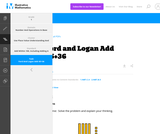Conditions of Use:
No Strings Attached
Rating

This is a task from the Illustrative Mathematics website that is one part of a complete illustration of the standard to which it is aligned. Each task has at least one solution and some commentary that addresses important aspects of the task and its potential use.

Subject:
Numbers and Operations
Material Type:
Activity/Lab
Provider:
Illustrative Mathematics
Provider Set:
Illustrative Mathematics
Author:
Illustrative Mathematics
02/16/2018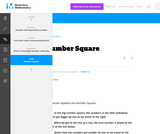Conditions of Use:
No Strings Attached
Rating

This is a task from the Illustrative Mathematics website that is one part of a complete illustration of the standard to which it is aligned. Each task has at least one solution and some commentary that addresses important aspects of the task and its potential use.

Subject:
Numbers and Operations
Material Type:
Activity/Lab
Provider:
Illustrative Mathematics
Provider Set:
Illustrative Mathematics
Author:
Illustrative Mathematics
02/16/2018Conditions of Use:
No Strings Attached
Rating

This is a task from the Illustrative Mathematics website that is one part of a complete illustration of the standard to which it is aligned. Each task has at least one solution and some commentary that addresses important asects of the task and its potential use. Here are the first few lines of the commentary for this task: Materials For each pair: * 2 ten-sided dice with the numbers 0 to 9 or two spinners with the numbers 0 to 9 * Base-10 blocks, linking cubes, or bundled...

Subject:
Mathematics
Material Type:
Activity/Lab
Provider:
Illustrative Mathematics
Provider Set:
Illustrative Mathematics
Author:
Illustrative Mathematics
05/24/2013Conditions of Use:
No Strings Attached
Rating

This is a task from the Illustrative Mathematics website that is one part of a complete illustration of the standard to which it is aligned. Each task has at least one solution and some commentary that addresses important asects of the task and its potential use. Here are the first few lines of the commentary for this task: Materials Set of cards from 1-100, these can be purchased or created using a black marker and index cards Setup Sort the 1-100 cards into sequential gr...

Subject:
Mathematics
Material Type:
Activity/Lab
Provider:
Illustrative Mathematics
Provider Set:
Illustrative Mathematics
Author:
Illustrative Mathematics
03/16/2013Conditions of Use:
Remix and Share
Rating

In Grade 5, students extend their understanding of the base-ten system to decimals to the thousandths place, building on their Grade 4 work with tenths andhundredths. They become fluent with the standard multiplication algorithm with multi-digit whole numbers. They reason about dividing whole numbers withtwo-digit divisors, and reason about adding, subtracting, multiplying, and dividing decimals to hundredths.(The Common Core State Standards Writing Team, 2012, p.16)The excerpt above, taken from the K-5, Number and Operations in Base Ten progression document, is a summary of what students should learn to do and understand by theendof Grade 5. While this summary provides a sense of the number and operations work at this grade, there are several essential ideas that students should engage with throughout their learning of number and operations across this school year. As such, this unit is the first of four units in Grade 5 that focus on developing students’ understanding of number and operations.Knowing that decimals follow the same base-ten structure as do whole numbers is a foundational concept for students to develop in this unit. With this knowledge students learn to apply strategies they used when working with whole numbers to their work with decimals, an idea that continues throughout later Grade 5 units. As the first of four Grade 5 units devoted to these ideas, students working in this unit explore decimals to the thousandths place. Their work includes reading, writing, rounding, and comparing decimals using a variety of notations (e.g. 167 x 100 = 16,700 = 167 hundreds = 167 x 10 x 10 = 167 x 10In Grade 4 students worked with multiples of ten. Since whole number exponents is a new representation and concept in Grade 5, students are likely to need support making connections between multiples of ten and powers of ten.

Subject:
Mathematics
Material Type:
Unit of Study
Provider:
OS/MAISA
03/22/2018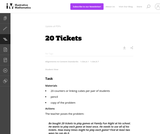Conditions of Use:
No Strings Attached
Rating

This is a task from the Illustrative Mathematics website that is one part of a complete illustration of the standard to which it is aligned. Each task has at least one solution and some commentary that addresses important asects of the task and its potential use. Here are the first few lines of the commentary for this task: Materials 20 counters or linking cubes per pair of students pencil copy of the problem Actions The teacher poses the problem: Bo bought 20 tickets to p...

Subject:
Mathematics
Material Type:
Activity/Lab
Provider:
Illustrative Mathematics
Provider Set:
Illustrative Mathematics
Author:
Illustrative Mathematics
05/05/2013Conditions of Use:
No Strings Attached
Rating

This is a task from the Illustrative Mathematics website that is one part of a complete illustration of the standard to which it is aligned. Each task has at least one solution and some commentary that addresses important asects of the task and its potential use. Here are the first few lines of the commentary for this task: Materials * A cup for each student to represent his/her cave * Counters * Recording sheet Actions The teacher begins by counting out a certain number o...

Subject:
Mathematics
Material Type:
Activity/Lab
Provider:
Illustrative Mathematics
Provider Set:
Illustrative Mathematics
Author:
Illustrative Mathematics
03/25/2014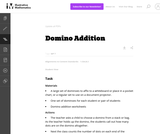Conditions of Use:
No Strings Attached
Rating

This is a task from the Illustrative Mathematics website that is one part of a complete illustration of the standard to which it is aligned. Each task has at least one solution and some commentary that addresses important asects of the task and its potential use. Here are the first few lines of the commentary for this task: Materials A large set of dominoes to affix to a whiteboard or place in a pocket chart, or a regular set to use on a document projector. One set of domi...

Subject:
Mathematics
Material Type:
Activity/Lab
Provider:
Illustrative Mathematics
Provider Set:
Illustrative Mathematics
Author:
Illustrative Mathematics